HSPT Quantitative : Non-Geometric Comparison

Example Questions

Example Question #71 : Non Geometric Comparison

Examine (a), (b), and (c) to find the best answer:

a)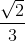b)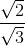c)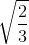(b) is equal to (c) but not (a)

(a), (b), and (c) are all unequal

(a), (b), and (c) are all equal

(b) is equal to (a) but not (c)

(b) is equal to (c) but not (a)

Explanation:

The property of exponents to remember here is that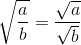.

This explains why (b) and (c) are equal. (a) cannot be equal because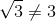.

Example Question #72 : Non Geometric Comparison

Examine (a), (b), and (c) to find the best answer:

a)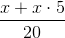b)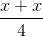c)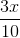(a), (b), and (c) are all unequal

(a) is equal to (c) but not (b)

(a) is equal to (b) but not (c)

(a), (b), and (c) are all equal

(a) is equal to (c) but not (b)

Explanation:

Following the order of operations, (a) simplifies to be (c):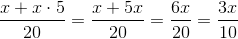(b) simplifies to the following: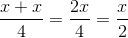Example Question #73 : Non Geometric Comparison

Examine (a), (b), and (c) to find the best answer:

a)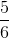b)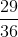c)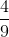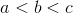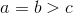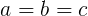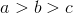Explanation:

Find a common denominator to compare the fractions. Here, we use:

a)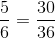b)c)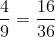It is now evident that (a) is greater than (b), which is greater than (c).

Example Question #74 : Non Geometric Comparison

Examine (a), (b), and (c) to find the best answer:

a)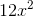b)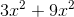c)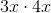(a) equals (b) but not (c)

(a), (b), and (c) are all unequal

(a), (b), and (c) are all equal

(a) equals (c) but not (b)

(a), (b), and (c) are all equal

Explanation:

(a) is a simplified version of both (b) and (c). For (b), you can add the like terms together and end up with (a). For (c), you can multiply the constants and the variables together and also end up with (a). To test this, try substituting a number in for.

Example Question #75 : Non Geometric Comparison

Examine (a), (b), and (c) to find the best answer:

a)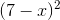b)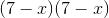c)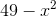(a) is equal to (c) but not (b)

(a), (b), and (c) are all unequal

(a), (b), and (c) are all equal

(a) is equal to (b) but not (c)

(a) is equal to (b) but not (c)

Explanation:

Test this problem by substituting a number in for. For example, here is:

a)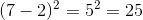b)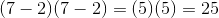c)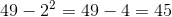It is evident that (a) and (b) are equal but (c) is not.

Example Question #76 : Non Geometric Comparison

Examine (a), (b), and (c) to find the best answer:

a)percent

b)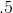c)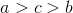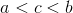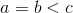Explanation:

Convert all of the values into decimals to compare them:

a)percent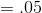b)c)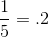It is now evident that (a) is smaller than (c), which is smaller than (b)

Example Question #77 : Non Geometric Comparison

Examine (a), (b), and (c) to find the best answer:

a)percent of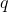b)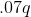c)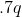(a) is equal to (c) but not (b)

(a), (b), and (c) are all unequal

(a) is equal to (b) but not (c)

(a), (b), and (c) are all equal

(a) is equal to (b) but not (c)

Explanation:

To find the percent of a number, in this case, multiply by the percent over one hundred: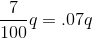Therefore, (a) and (b) are equal.would bepercent.

Example Question #78 : Non Geometric Comparison

Examine (a), (b), and (c) to find the best answer:

a)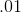percent ofb)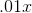c)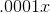(a) is equal to (c) but not (b)

(a) is equal to (b) but not (c)

(a), (b), and (c) are all unequal

(a), (b), and (c) are all equal

(a) is equal to (c) but not (b)

Explanation:

To find a percentage of the number, multiply it by the decimal version of the percent, or the percent divided by.

Therefore,percent ofis equal to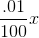, orExample Question #79 : Non Geometric Comparison

Exmaine (a), (b), and (c) to find the best answer:

a)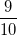ofb)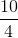ofc)ofExplanation:

Multiply out each expression to compare the values:

a)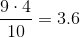b)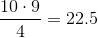c)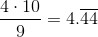It is now evident that (a) is smaller than (c), which is smaller than (b)

Example Question #80 : Non Geometric Comparison

Examine (a), (b), and (c) to find the best answer:

a)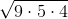b)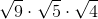c)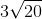(a), (b) and (c) are all unequal

(a) is equal to (c) but not (b)

(a), (b) and (c) are all equal

(a) is equal to (b) but not (c)

(a), (b) and (c) are all equal

Explanation:

You don't need to calculate any square roots to solve this problem! Just remember the following property: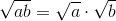Following this property, (a) and (b) are equal: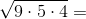This also means that the following is true: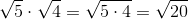And therefore: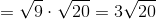(c) is just a simplified version of (a) and (b)